3. The Cosmological redshift

One observable prediction of an expanding universe is that of redshifting. When a light wave is traveling from a distant galaxy, to our own, it must travel through the intervening spacetime. This results in a stretching of the wavelength of light, since the spacetime is expanding. This longer wavelength results in the light being shifted to a `redder' part of the spectrum. Of course light with wavelengths differing from visible light will not be visible to the human eye, but they will still be shifted to longer wavelengths.

To quantify this analysis, consider a light ray which must travel along a null geodesic (ds2 = 0) in the comoving frame with constant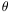and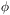. Using (4),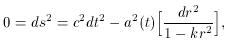so,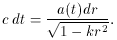Integrating yields,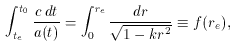(6)

where te is the time the light pulse was emitted, t0 was the time the light pulse was received, and re is the distance to the galaxy. Thus, if one knows a(t) and k, one can find the relation between the distance and the time. However, consider emitting successive wave crests in such a brief time that a(t) is not given a chance to increase by a significant amount; i.e., the waves are sent out at times te and te +te and received at times t0 and t0 +t0, respectively. Then (6) becomes,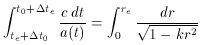Subtracting (6) from this equation and using the fact a(t) doesn't change, one can use the fundamental theorem of calculus to obtain,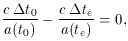or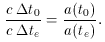ct is just the wavelength,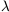. Thus, it follows that the red shift, z, can be defined by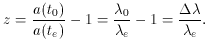Here a(t0) is the scale factor of the universe as measured by a comoving observer when the light is received, a(te) is the scale factor when the light was emitted in the comoving frame,0 is the wavelength observed ande is the wavelength when emitted. It is clear that z will be positive, since a(t0) > a(te), that is the universe is getting larger.

In addition to this cosmological redshift, which is due to the expanding universe, there can also be gravitational redshifts and Doppler redshifts. At great distances the former two can be neglected, but in local cases all three must be considered.

It must also be stressed that the Special Relativity (SR) formula for redshift can not be used. This is because SR only holds for `local' physics. Attempting to use this across large distances can result in a contradiction. For example, the expansion rate of the universe can actually exceed the speed of light at great distances. This is not a violation of SR, because a `chain' of comoving particles (galaxies) can be put together, spaced so the laws of SR are not violated. By summing together the measurements of each set of galaxies, one finds the expansion rate to exceed that of light, although locally SR holds locally . Another explanation is that in a universe described by SR, no matter or energy exists and the metric never changes. On the contrary, in an expanding spacetime none of these requirements are true. Although, SR continues to hold locally, since a `small enough' region can always be chosen where the metric is approximately flat. (12)

12 One must be careful by what is meant by `small enough'. This technical point need not concern us with the present discussion, see . Back.